# Ordered Dynamics in Genetic Networks

Steve E. Harris, Bruce Sawhill, Andrew Wuensche, Stuart Kauffman.

These plots were made for random Boolean networks, n=1000, for k=3, 4, and 5, using the old+new data. The C values are higher in all cases.
k=3, C=0.795
k=4, C=0.708
k=5, C=0.649
There are two diagrams for each k.

left: an overall plot showing rules at random, and with canalizing set. The normalized Hamming range is 0 to 0.5. Each Hamming distance is plotted for a sample of 25 initial pairs of states.

right: details of the initial Hamming range (0 to 0.01) to calculate the Hamming coefficient based on the first 5 steps. The average slope between these points and the origin is taken as the initial slope. If the initial slope = x degrees, then the Derrida coefficient, Dc=log2(tan(x)). A slope of 45 degrees (Dc=0) indicates the dynamics is balanced between order and chaos. A slope above the 45 degree diagonal (positive Dc) is in the chaotic regime, below (negative Dc) is in the ordered regime. If Derrida plot is based on more than 1 iteration, the slope above or below the diagonal is amplified, giving a clear indication where it lies relative to the 45 degree diagonal.
The plots how 4 curves. The top curve is for rules set at random. The 3 lower curves are for canalizing set, for 1, 2 and 3 iterations. Each Hamming distance is plotted for a sample of 1000 initial pairs of states. These are typical plots, and the variance for other networks with the same parameters was found to be low.

Comments: the curves no longer lie on each other, but become shallower with greater k. The k=3 curve in the old paper looks too shallow compared with new k=3 curve, which is steeper despite having greater C.

Click on each image to see it full size.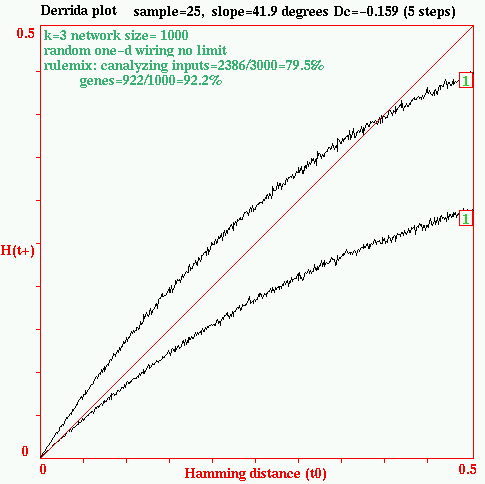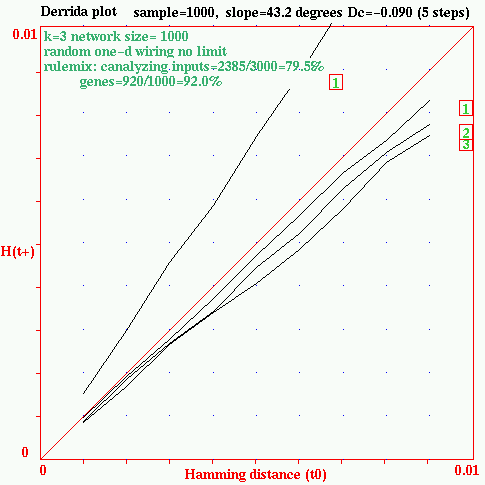k=3, n=1000. Steep curve: random rules. Shallow curve: C=0.795. Derrida coefficient. Steep curve: random rules, Dc=+0.58 Shallow curve, 1 itteration, Dc=-0.9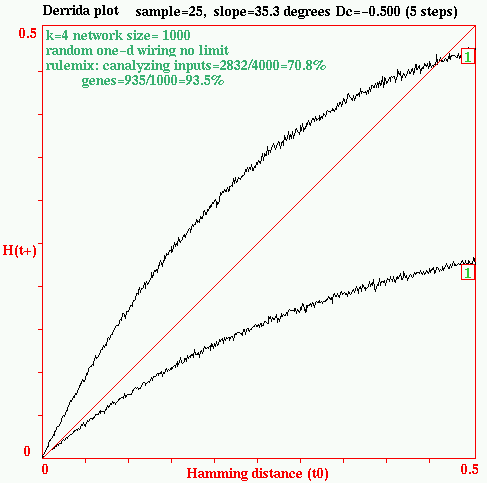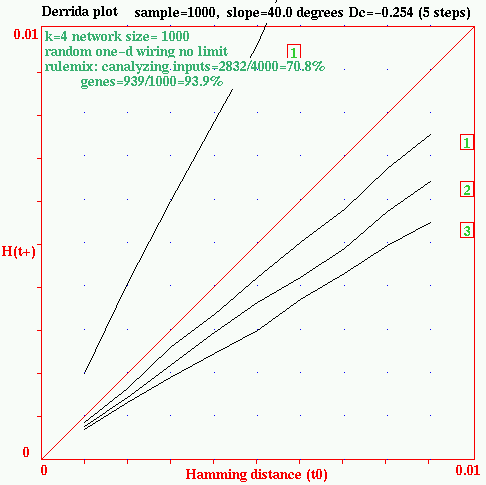k=4 n=1000. Steep curve: random rules. Shallow curve: C=0.708. Derrida coefficient. Steep slope: random rules, Dc=+1.31 Shallow curve, 1 itteration, Dc=-0.25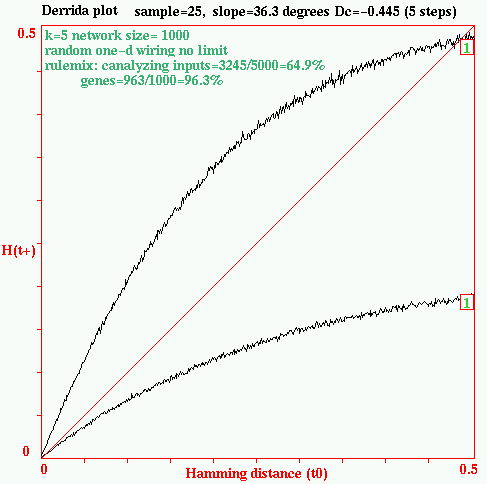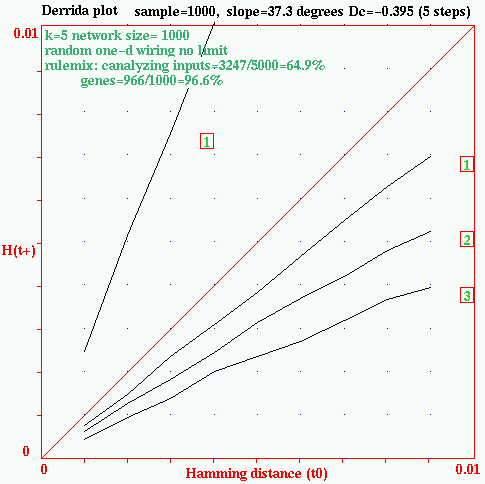k=5 n=1000. Steep curve: random rules. Shallow curve: C=0.649. Derrida coefficient. Steep curve: random rules, Dc=+1.33 Shallow curve, 1 itteration, Dc=-0.39